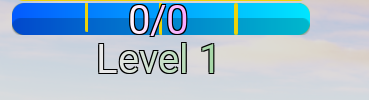# Need help with using datastores without leaderboards

Hello,

I’ve been working on an RPG game, and I have a leveling system I made that works, but I am wondering, stuck on trying to make it save. I’ve been trying to make this system save for 2 hours, but I just can’t do it.

I would like to save these values without using a leaderboard system, is that possible?

Here is my leveling script:

``````game.Players.PlayerAdded:Connect(function(player)
local level = Instance.new("IntValue", player)
level.Name = "Level"
level.Value = 1

local exp = Instance.new("IntValue", level)
exp.Name = "Current"
exp.Value = 0

local maxExp = Instance.new("IntValue",level)
maxExp.Name = "Max"
maxExp.Value = 100

exp.Changed:Connect(function(val)
if exp.Value >= maxExp.Value then
level.Value = level.Value + 1
exp.Value = 0
maxExp.Value = maxExp.Value * 1.5
end
end)
end)
``````
1 Like

You do it exactly the same as a leaderboard, if you don’t want to use a leaderboard you can for example create a folder, name it “Data” and then store everything there instead

2 Likes

Would that still work if the values are stored inside Game > Players > (PlayerName)?

1 Like

Yes, Roblox data store’s content are not instances, they are just values. You can save numbers like 0,1,2,3, tables, text etc… and it will be the same. In the leaderboard it is the same, there are values inside a folder ofc, but you store the data by getting those values. Moving them from place won’t matter

I tried doing that, and it broke the leveling system

``````local DataStoreService = game:GetService("DataStoreService")
local myDataStore = DataStoreService:GetDataStore("myDataStore")

local Level = Instance.new("IntValue")
Level.Name = "Level"
Level.Parent = player

local leveldata
local success, errormessage =pcall(function()
leveldata = myDataStore:GetAsync(player.UserId.."-Level")
end)

if success then
Level.Value = leveldata
else
warn(errormessage)
end

end)

game.Players.PlayerRemoving:Connect(function(player)

local success, errormessage = pcall(function()
myDataStore:SetAsync(player.UserId.."-Level",player.Level.Value)
end)

if success then
print("Player Data Saved!")
else
print("Error when saving!")
warn(errormessage)
end

end)
``````

This was the saving system I made
I think the reason why its not working is because of this part in the leveling script,

``````	exp.Changed:Connect(function(val)
if exp.Value >= maxExp.Value then
level.Value = level.Value + 1
exp.Value = 0
maxExp.Value = maxExp.Value * 1.5
``````

How would I merge the two scripts together?

Is there an error that made it broke?

When I join the game, the leveling system doesnt work anymore, I dont think they’re compatible.

The scripts mess with each otherNormally, it would say 0/100, but when I enable the saving script, it breaks, and now you cant gain XP

Level here is lowercase meanwhile you have set your variable to “Level”

That is just the name of that line of code, the real value name is uppercase

You should be able to merge them normally. Add a print int he .Changed event to print when the value changes. Does it print?

I made a saving system, and I have a question

``````local DataStoreService = game:GetService("DataStoreService")
local playerData = DataStoreService:GetDataStore("PlayerData")

local function onPlayerJoin(player)

local Level = Instance.new("IntValue")
Level.Name = "Level"

local EXP = Instance.new("IntValue")
EXP.Name = "XP"

local MaxEXP = Instance.new("IntValue")
MaxEXP.Name = "MaxXP"

local playerUserId = "Player_"..player.UserId
local data = playerData:GetAsync(playerUserId)
if data then
Level.Value = data["Level"]
EXP.Value = data["XP"]
MaxEXP.Value = data["MaxXP"]
else
Level.Value = 0
EXP.Value = 0
MaxEXP = 0
end
end

local function create_table(player)
local player_stats = {}
for _, stat in pairs(player.leaderstats:GetChildren()) do
player_stats[stat.Name] = stat.Value
end
return player_stats
end

local function onPlayerExit(player)
local player_stats = create_table(player)
local success, err = pcall(function()
local playerUserId = "Player_"..player.UserId
playerData:SetAsync(playerUserId, player_stats)
end)

end

game.Players.PlayerRemoving:Connect(onPlayerExit)
``````

For the leveling system to actually level up, it needs this:

``````	exp.Changed:Connect(function(val)
if exp.Value >= maxExp.Value then
level.Value = level.Value + 1
exp.Value = 0
maxExp.Value = maxExp.Value * 1.5
``````

Where would I put that? Would I put that at the very bottom?

``````if data then
Level.Value = data["Level"]
EXP.Value = data["XP"]
MaxEXP.Value = data["MaxXP"]
else
Level.Value = 0
EXP.Value = 0
MaxEXP = 0
end
``````

After that

Is this correct?

``````	if data then
Level.Value = data["Level"]
EXP.Value = data["XP"]
MaxEXP.Value = data["MaxXP"]
else
Level.Value = 0
EXP.Value = 0
MaxEXP = 0
end

EXP.Changed:Connect(function(val)
if EXP.Value >= MaxEXP.Value then
Level.Value = Level.Value + 1
EXP.Value = 0
MaxEXP.Value = MaxEXP.Value * 1.5
end
``````

Do I need to add any more spaces between code or is it all good?

well you need the "end"s, 2 of them

Like this?

``````	else
Level.Value = 0
EXP.Value = 0
MaxEXP = 0
end

EXP.Changed:Connect(function(val)
if EXP.Value >= MaxEXP.Value then
Level.Value = Level.Value + 1
EXP.Value = 0
MaxEXP.Value = MaxEXP.Value * 1.5
end
end)
``````
``````EXP.Changed:Connect(function(val)
if EXP.Value >= MaxEXP.Value then
Level.Value = Level.Value + 1
EXP.Value = 0
MaxEXP.Value = MaxEXP.Value * 1.5
end
end);``````

Okay thanks. I have one last question though, at the very bottom of the script, there is a red line under (onPlayerExit). Is that going to be an issue?Hover over it, what does it say?

It says it expected ends to lines 4 and 51, but there are ends there.